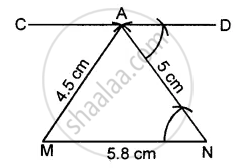# Draw a Line Mn = 5.8 Cm. Locate a Point a Which is 4.5 Cm from M and 5 Cm from N. Through a Draw a Line Parallel to Line Mn. - Mathematics

Sum

Draw a line MN = 5.8 cm. Locate a point A which is 4.5 cm from M and 5 cm from N. Through A draw a line parallel to line MN.

#### Solution

Steps of construction :

1. Draw a line MN = 5.8 cm
2. With M as centre and radius = 4.5 cm, draw an arc.
3. With N as centre draw another arc of radius 5 cm. These arcs intersect each other at A.
4. Join AM and AN.
5. At point A, draw ∠DAN = ∠ANM
6. Produce DA to any point C.
Thus CAD is the required parallel line.Concept: Construction of an Angle - Construction of Parallel Lines
Is there an error in this question or solution?

#### APPEARS IN

Selina Concise Mathematics Class 8 ICSE
Chapter 18 Constructions
Exercise 18 (C) | Q 2 | Page 205